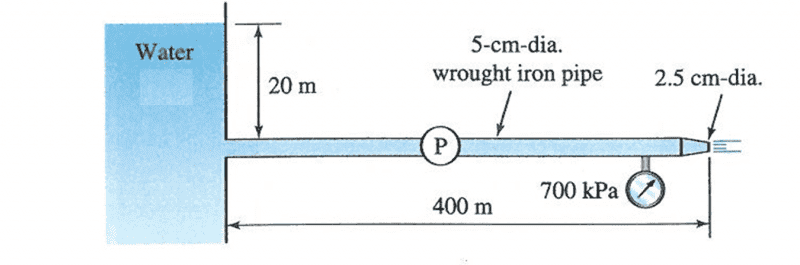# Calculate fluid flow in pipeline

## Homework Statement

The pump in the system below has an efficiency of 75%. The atmospheric pressure is 101 kPa and the vapor pressure is 2.3 kPa (in absolute units). Assume kinematic viscosity is 0.96x10-6 m2/s. Neglect minor losses.

a) Calculate the velocity through the main pipe and of the jet

and a few other questions to do with friction factor, etc.## Homework Equations

AV=AV

v^2/2g + P/pg = v^2/2g + P/pg

Have been given the answers but no working. – 9.66 m/s for pipe and 38.64 m/s for jet

## The Attempt at a Solution

First used AV=AV to get

v_2 = [(d_1)^2/(d_2)^2] v_1

Then I put this into Bernoulli with the relevant pressures P_1 = 700000, P_2 = 101000 but I only manage to get v_1 = 8.94 m/s.

I have tried working it with elevation head but still can't seem to get the right answer. I am thinking maybe I should have pump head? But not too sure how to calculate with what I have been given.

## Answers and Replies

Simon Bridge
Science Advisor
Homework Helper
My immediate thought is that 700kPa is a gauge pressure.
The 20m head provides pressure at the other end of the pipe - which should tell you what the pump is doing.

It may help if you think of the pump as replacing a drop in height.

Chestermiller
Mentor
You mentioned in your original post the "friction factor", and one of the pieces of data given was the kinematic viscosity. Where were these in your calculations? That pipe is only 2" in diameter, and is 400 meters long. Do you really think that the Bernoulli equation is going to give you an accurate prediction for this problem? What have you left out?

Chet

My immediate thought is that 700kPa is a gauge pressure.
The 20m head provides pressure at the other end of the pipe - which should tell you what the pump is doing.

It may help if you think of the pump as replacing a drop in height.

Thanks that helped get it sorted, changed my P_2 value and found the right answer

You mentioned in your original post the "friction factor", and one of the pieces of data given was the kinematic viscosity. Where were these in your calculations? That pipe is only 2" in diameter, and is 400 meters long. Do you really think that the Bernoulli equation is going to give you an accurate prediction for this problem? What have you left out?

Chet

They are parts b,c,d when they want the friction factor, pump power and distance from reservoir to avoid cavitation. I can't seem to solve the NPSM but will keep trying.

Chestermiller
Mentor
Yes. I looked over the problem again, and Simon was right regarding the gage pressure (as your answer confirms). You next need to calculate the Reynolds number for the flow in the pipe. This will then allow you to determine the friction factor.

chet